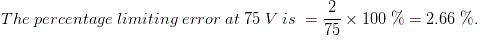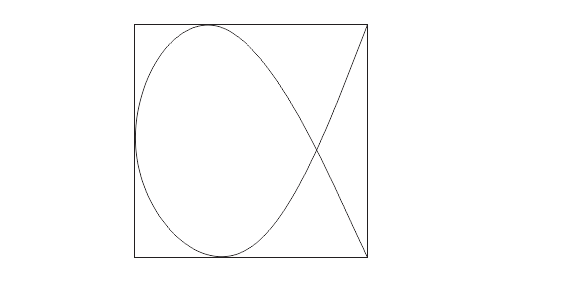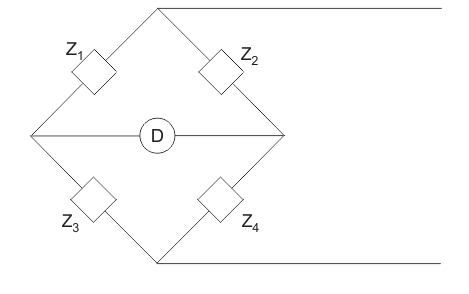# MCQs on Electrical And Electronics Measurements

##### Page 7 of 19. Go to page 1 2 3 4 5 6 7 8 9 10 11 12 13 14 15 16 17 18 19
01․ Relative error is same as
absolute error.
true error.
ratio of absolute error and true value.
none of the above.

Relative error is the ratio of absolute error to the true value of the unknown quantity to be measured.

02․ A 0 - 100 V voltmeter has a guaranteed accuracy of 2 % of full scale reading. The voltage measured by the voltmeter is 75 V. The limiting error is in percentage
3.33 %.
2.66 %.
2 %.
1 %.

The magnitude of the limiting error of the instrument = relative error X scale division = 0.02 X 100 = 2 V.03․ The moving coil-meters, damping is provided by
the aluminium frame of the coil.
the coil spring attached to the moving.
eddy current disk.
damping vane in the airtight chamber.

The movement induces emf and hence current flows in the aluminium frame of the coil. The torque developed due to this current is the damping torque.

04․ A lissajous pattern, as in figure is observed on the screen of a CRO when voltage of frequencies fx and fy are applied to the X and Y plates respectively. Then fx:fy is then equal to1:2.
2:1.
3:2.
2:3.

fx/fy = points of tangency to vertical line/points of tangency to horizontal line = 1/2.

05․ In figure Z1 = 200∠60° ohm. Z2 = 400∠-90° ohm, Z3 = 300∠0° ohm. Then Z4 for bridge to be balanced is150∠30° ohm.
400∠-90° ohm.
300∠90° ohm.
600∠-150° ohm.

For balanced condition, Z1Z4 = Z2Z3.

06․ The coil of a moving coil meter has 100 turns, is 40 mm long and 30 mm wide. The control torque is 240×10-6 N-m on full scale. If magnetic flux density is 1Wb/m2 range of meter is
1 mA.
2 mA.
3 mA.
4 mA.

In case of moving coil meter, Controlling torque Tc = NBIA. Where, N = no. of turns of the coil, B - magnetic flux density, = A = area of coil. So, I = 240×10-6 / (100 × 0.04 × 0.03) = 2mA.

07․ The scale of hot wire ammeter is
Linear.
Non Linear.
Cramped.
Absloute linear.

In hot wire ammeter, the deflecting torque is proportional to the square of the rms value of operating current. Hence scale is non linear.

08․ The resistance in the circuit of the moving coil of a dynamometer wattmeter should be
Low.
Very low.
High.
Almost zero.

In dynamometer wattmeter, the moving coil is voltage coil. So the moving coil must have high resistance.

09․ The dynamometer principle can be used for
ammeter only.
wattmeter only.
voltmeter only.
ammeter, voltmeter and wattmeter.

Dynamometer principle can be used for ammeter, voltmeter and wattmeter. However it is generally used for wattmeter only.

10․ A Lissajous pattern on an oscilloscope has 5 horinzontal tangencies and 2 vertical tangencies. The frequency of horizontal input is 100 Hz. The frequency of the vertical will be
500 Hz.
250 Hz.
400 Hz.
625 Hz.

fx /fy = points of tangency to vertical line/points of tangency to horizontal line = 1/2.

<<<56789>>>